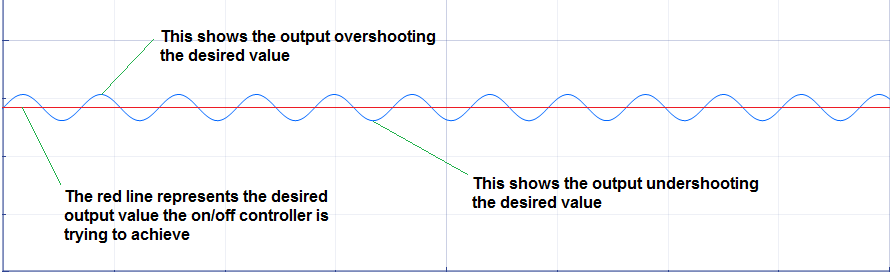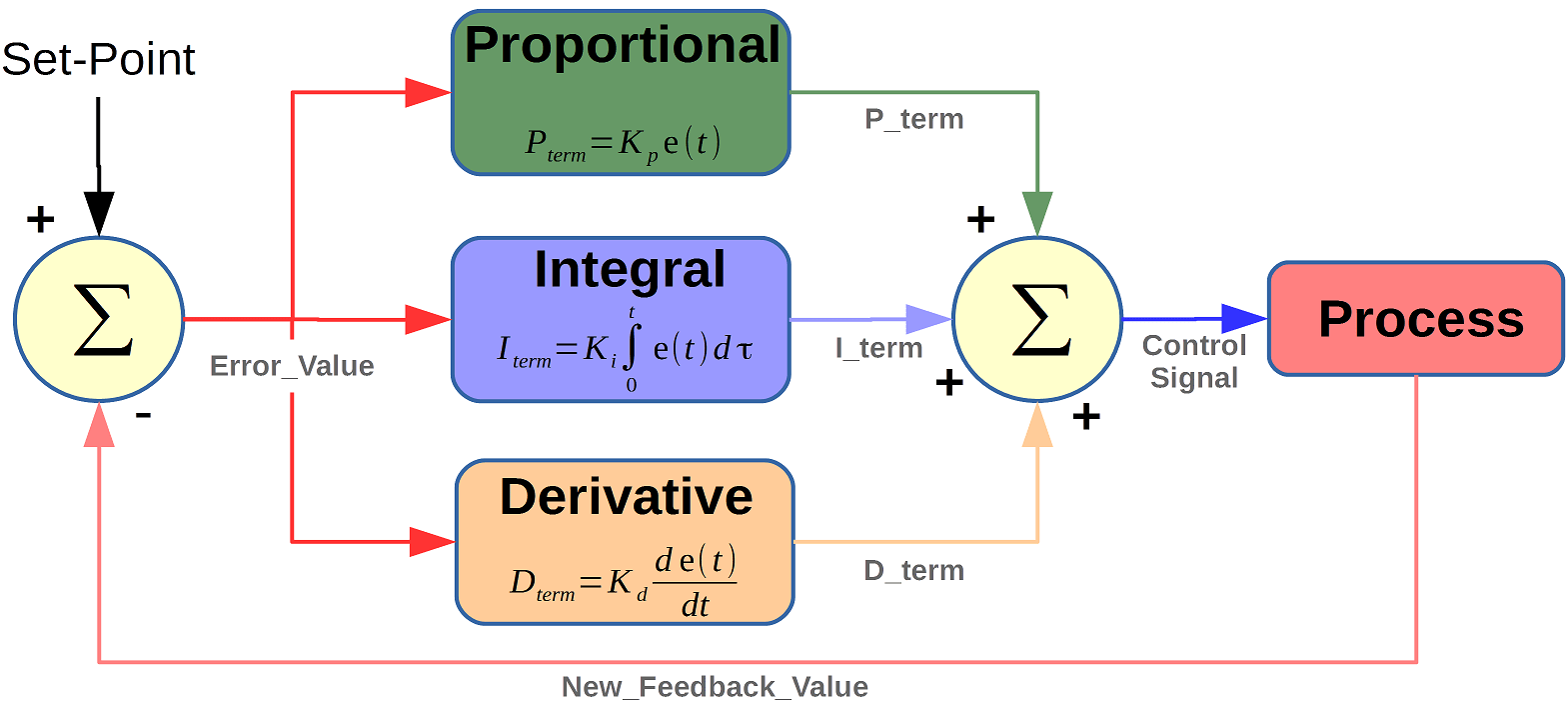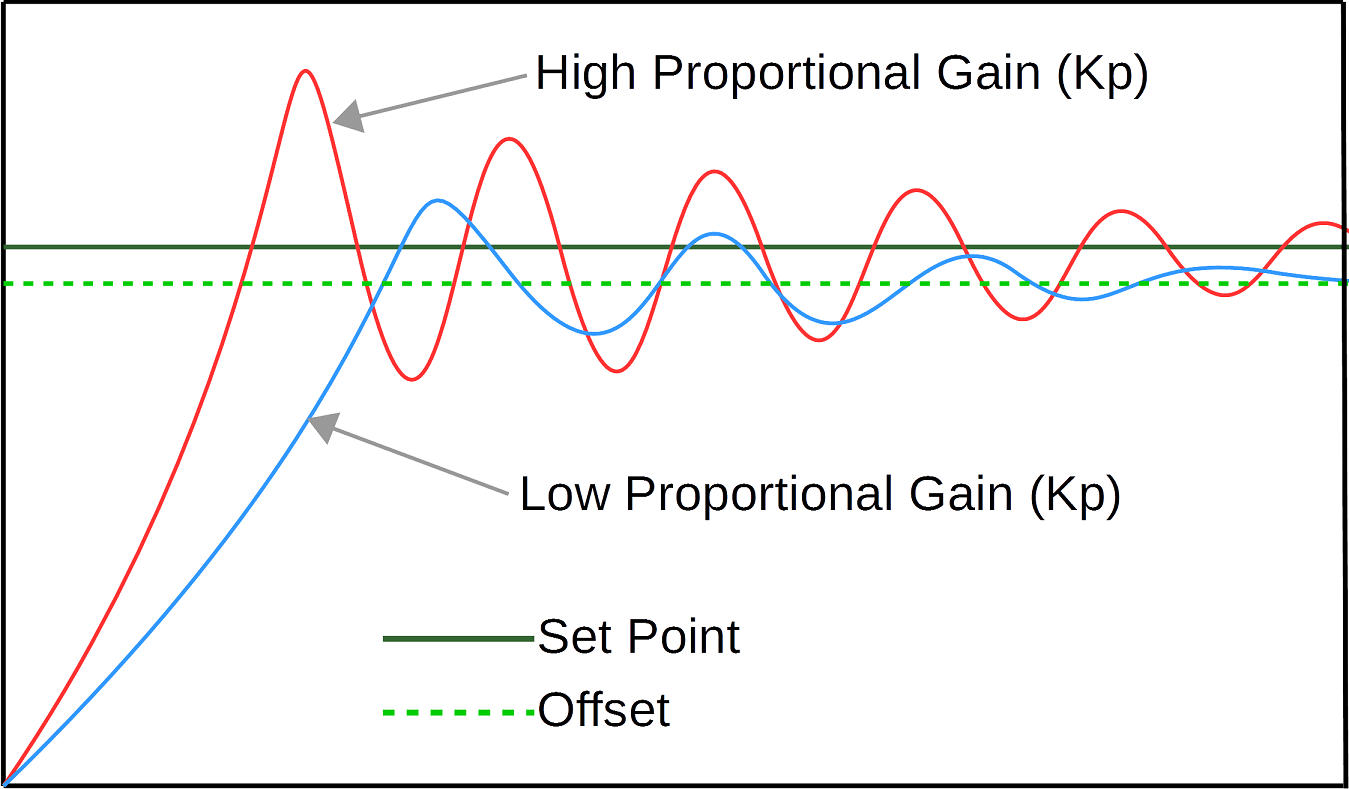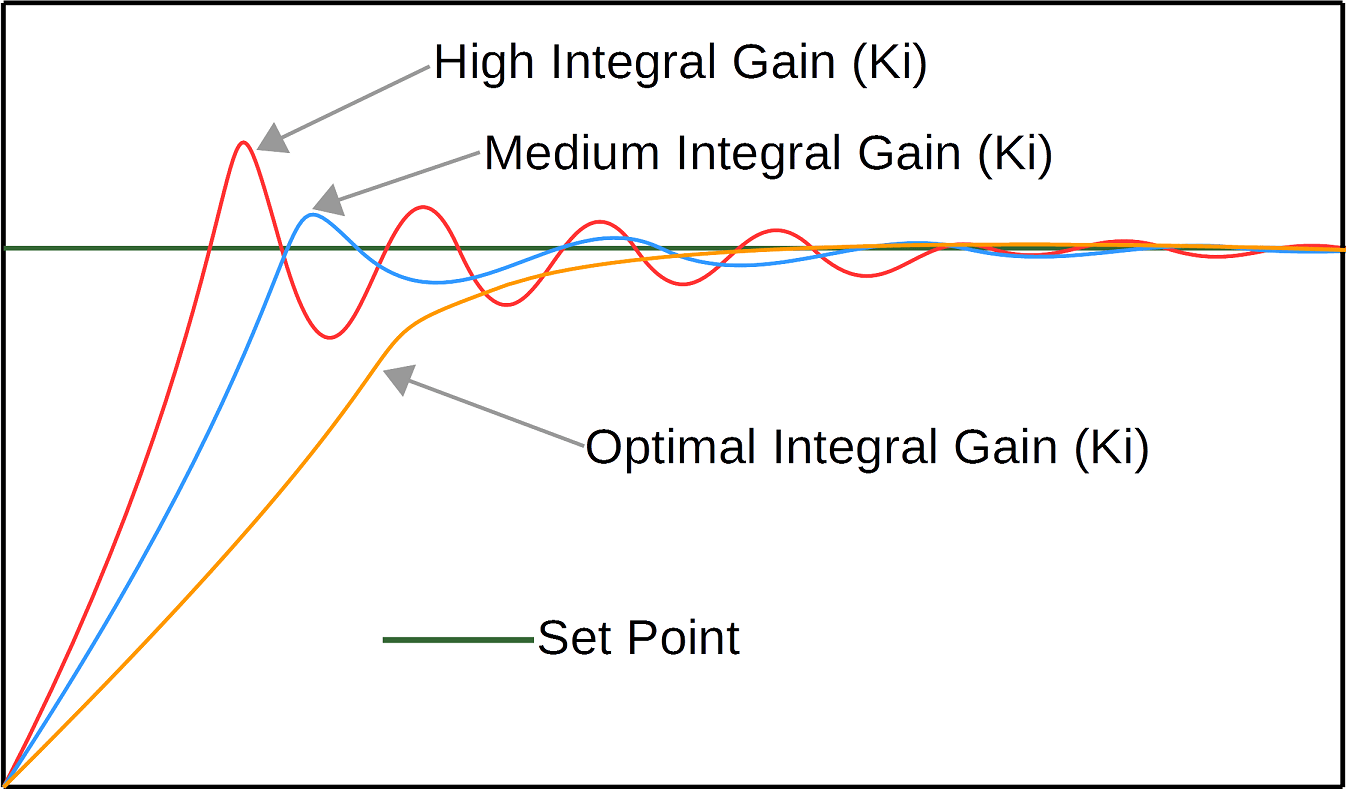# PID Tutorial C code Example Pt/1

In this PID tutorial C code example Pt/1, the basic concept of a PID controller will be explored. The full example code is implemented on a Stellaris LM3S6965 microcontroller, and is used to control a simple boost converter circuit, regulating the voltage with a change in load resistance. The boost circuit is not covered in-depth in this tutorial, but the fundamentals of a boost circuit are covered.

In this first part the basic concepts of a control system will be explored, as well as the PID controller.  The second parts covers the code implementation in the C language on the Stellaris LM3S6965, all the code is stepped through and can be downloaded at the end of the second part.

## Basic Control System Concepts

To understand how PID controllers are used, it is important to first understand some basic control system principles.  The image below illustrates the 2 basic types of control system.The open loop system can clearly be seen to have no feedback, therefore if the load changes on the motor, the motor speed will change.  The control unit cannot command the driver to increase or decrease the power to the motor, as it has no knowledge of the speed change induced in the motor, by the change in load.

The closed loop system however has feedback from the motor.  So if the motors speed were to decrease due to an increase in load, the control unit could command the driver to increase the power to the motor, keeping a constant speed.  Common direct current motor control is achieved via PWM, and simply increasing or decreasing the duty cycle will increase or decrease the motor speed.

## On/Off Controller

Using the above closed control loop as an example, the basic operation can be broken down. The feedback signal will often go through some signal conditioning circuitry, before being fed to the control unit.  The control unit will have some reference set point or threshold levels, that it is aiming to keep the motor speed at or between.  If a reference set point is used the new feedback signal could be used to generate an error value in the following way

Error_Value = Set_Point – New_Feedback_Value

We will come back to the Error_Value shortly as this is used in the PID controller, but first lets look at a more simplistic approach using the threshold level.

If threshold levels are used, lets say to keep the motor speed between 1200-1300rpm, the feedback signal could be directly used as a comparison.  Therefore if the feedback signal is 1320rpm the control unit needs to decrease the motor speed.  If we now said the control unit was a microcontroller, and the driver was a simple amplifier.  The microcontroller could reduce the duty cycle of the PWM, this in turn would then slow the motor down.  If we consider the motor to have a very quick response to changes in PWM drive, and the load on the motor to alter subtle over time, then there are 2 important factors to consider.  (1) The speed at which the PWM duty cycle is updated by the microcontroller, and (2) how large a change is made to the duty cycle on each update i.e. 1%, 5% etc.  This control system is often known as an on/off controller, the 2 factors can be tuned to suit the individual control system.  An example of on/off controller which is slightly more complex than this example, as it has other parameters is a Perturb and Observe algorithm used in a solar MPPT for photovoltaics.  The image below shows how an on/off controller usually oscillates around the set output level.On/off control systems typically oscillate around the desired output level, as they overshoot and undershoot the desired value.  The threshold level example used has no fixed setpoint so even if the motor speed stayed within these bounds, its speed would still oscillate to some degree.  The size of these oscillations is dependant on the PWM step size and the control loop speed.  If the system is very dynamic and changing at a fast rate, then a compromise between these 2 factors needs to be reached, to ensure good tracking performance.  When the desired reference value is reached, the size of the oscillations will be dependant on the PWM step size.

## PID Controller

PID stands for Proportional, Integral and Derivative.  PID controllers have been used since the 1890s, and were originally developed by Elmer Sperry for an automatic ship steering system.  A PID control system uses 3 gain constants, which are multiplied with the Error_Value.  The proportional value gain constant is referred to as Kp, the integral value gain constant as Ki and the derivative value gain constant as Kd.   The results of these multiplications produces 3 further values, for this tutorial these are referred to as the P_term, I_term and D_term.

As previously mentioned the Error_Value can be obtained from simply taking the New_Feedback_Value away from the Set_Point.  In a PID controller the error value can simply be multiplied with the Kp constant, but for the Ki and Kd constants a different approach is required.  In order to calculate the I_term and D_term historic error values are required.  The I_term is using integration which gives the average error value over time, and the D_term is using differentiation which gives the rate of change of the error value over time.  Once all the PID terms are calculated, these new values are then summed together and used in the final control output.

The P, I and D terms have different affects on the final system performance, and need to be tuned to the individual control system.  The ultimate aim for any control system is to respond as quickly as possible, without excessive overshoot and oscillations around the set point.  The system response ideally needs to reach a settled state as quickly as possible, the settled state is very subjective and dependant on the application.  A block diagram of a PID control system can be seen below.The proportional term (P_term) provides an output value which is proportional to the current error value.  The Kp constant value determines how large the gain response will be to the current error value.  A high proportional gain constant will induce a large change in the output, often overshooting the set point level.  If the gain is too high the system can become unstable and excessive oscillations can occur.  Another affect of a high Kp constant is faster response time, vice versa a low Kp constant will result in a slower response time.  When a low Kp constant is used, there will still be oscillations and some overshoot of the set point depending on the level, however these will not be as aggressive.

A low Kp value will also settle in an offset position slightly below the set point level, this offset position can be used when tuning a PID system.  The image below illustrates the step response waveforms of a system with high and low Kp gain constants, on a hypothetical control system.The integral term (I_term) is used to bring long term precision to the control loop.  It is proportional to both the magnitude and duration of the error.  Using Integration allows for the average error to be calculated, as the sum of the instantaneous error over time provides an accumulated offset.  The accumulated error is then multiplied by the Ki gain constant, allowing the offset which should have been corrected previously, to be added to the controller output.  This then accelerates the controller output response towards the set point, eliminating the offset found when just using a proportional control value.

As with the the Kp gain constant the Ki constant, needs to be tuned.  If the Ki value is too high the system will have a faster response time, but it will also be likely to overshoot the set point, inducing further unwanted oscillations before finally settling.  An ideal Ki value produces a response time that is fast enough for the application, with little or no overshoot and a quick settling time.  The image below illustrates the step response waveforms with a tuned Kp value already set, then a few Ki gain constants are applied on a hypothetical control system.The derivative term (D_term) is the least used of the three terms, most controllers are based on PI control algorithms.  Introducing the Kd term is generally used in specific control systems, these are usually where overshooting the set point can cause dangerous results. Differentiation is used to determine the rate of change, by using the instantaneous error value and the previous error value, so a rate of change can be determined.  The derivative term can be used to predict system behaviour, improving settling time.

## PID Control Loop Tuning

As can be seen the constant gain values Kp, Ki and Kd all affect the control system in different ways, therefore careful tuning of these values is an essential part of an effective PID controller.  Tuning a PID system is a difficult problem, there are only 3 parameters but finding a balance often takes some time depending on the complexity of the system.  Some systems have auto tuning features, but in some situations to have the best performance from a system, it is often recommended to manually tune.

A well known tuning method is called Ziegler-Nichols, developed by John G. Ziegler and Nathaniel B. Nichols.  This relies on the Ki and Kd gain constants first being set to zero.  The the Kp constant is adjusted until it reaches the ultimate gain, which is classified by the output from the control loop oscillating at a constant amplitude.  Then the ultimate gain value and oscillation period are used to calculate the Ki and Kd constants.  More about the Zieglar-Nichols method can be found here.

There are other methods and simply searching on Google will yield many results.

The C code, set-up and tuning of the boost converter will be covered in part 2 of this tutorial.

## 4 thoughts on “PID Tutorial C code Example Pt/1”

1.Abdelazez says:

Good job

1.Ant says:

Thanks

2.mahmoud says:

U help me a lot …thank U bro .

1.Ant says:

No worries